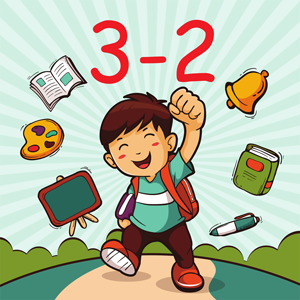This app has a free trial\$2.49
Overview System Requirements Related

## Description

Math Subtraction is a math learning app designed to help you to practice subtraction. The built-in handwriting recognition allows you to draw answers directly on the screen. Comes with a number of simple mini-games to make learning more effective and less boring. You can practice the following math skills: Subtraction facts - numbers up to 10 Subtraction facts - numbers up to 18 Subtract a multiple of ten Relate addition and subtraction Subtraction facts - numbers up to 20 Subtract a one-digit number from a two-digit number Subtract two two-digit numbers Subtract multiples of 10 Subtract multiples of 100 Subtract multiples of 10 or 100 Balance subtraction equations Subtraction facts - numbers up to 100 Subtract two three-digit numbers Complete the subtraction sentence up to three digits Subtract numbers with four or five digits Balance subtraction equations up to three digits

## Screenshots

Sergey Malugin

Sergey Malugin

5/26/2020

33.98 MB

For all ages

#### This app can

Access all your files, peripheral devices, apps, programs and registry
Microsoft.storeFilter.core.notSupported_8wekyb3d8bbwe

#### Installation

Get this app while signed in to your Microsoft account and install on up to ten Windows 10 devices.

#### Language supported

English (United States)